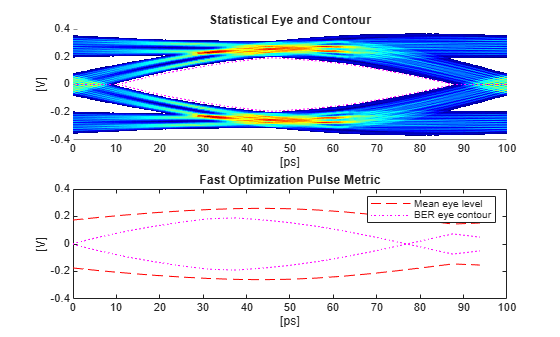# optPulseMetric

Pulse response metric for optimization routines

## Syntax

``metric = optPulseMetric(P,N,dt,B)``

## Description

example

````metric = optPulseMetric(P,N,dt,B)` calculates the eye height, eye width, eye area, and channel operating margin (COM) metrics at the target bit error rate (BER) level `B`. The function calculates these metrics from the pulse response matrix `P`, using the number of samples per symbol `N`, and the uniform sampling interval `dt`.If there is no eye at the target BER level `B`, then the `optPulseMetric` function increases the BER until a positive height is realized.The resolution of the `optPulseMetric` function is limited by number of samples per symbol `N`.The nonreturn to zero (NRZ) eye metrics are estimated at a BER level, where both the center and maximum eye heights are reported. If the eye is not open at the target BER, the BER is increased until a measurable eye contour is found. The actual BER used is reported by the value `usedBER` reported in the output `metric`.```

## Examples

collapse all

Define the system exploration parameters. Set the target bit error rate (BER) to 1e-9, symbol time to 100 ps, number of samples per symbol to 16, and channel loss to 4 dB.

```targetBER = 1e-9; SymbolTime = 100e-12; N = 16; dBLoss = 4;```

Calculate the sample interval.

`dt = SymbolTime/N;`

Create the channel model at the specified loss.

```channelModel = serdes.ChannelLoss('Loss',dBLoss,'dt',dt,... 'TargetFrequency',1/SymbolTime/2);```

Define a CTLE System object™.

```ctle = serdes.CTLE('WaveType','Impulse',... 'SampleInterval',dt,'SymbolTime',SymbolTime,'Mode',2);```

Process the channel impulse response with the CTLE System object.

`impulse = ctle(channelModel.impulse);`

Convert the impulse response to a pulse response.

`P = impulse2pulse(impulse,N,dt);`

Calculate the fast optimization pulse metric.

`metric = optPulseMetric(P,N,dt,targetBER);`

Obtain the result of the `optPulseMetric` function throughout the whole eye.

In order to do that, first find the number of complete unit intervals in the pulse response.

```nUI = floor(size(P,1)/N); prMatrixSort = sort(abs(reshape(P(1:N*nUI),N,[])).',1,'descend');```

Then convert the BER into the number of largest pulse response samples to get the `usedBER` contour. The mean height is the first row (largest value) of the sorted pulse response. The noise is the sum of sorted pulse response's next `nBER` sample intervals.

```nBER = floor(min(abs(log(targetBER)/log(2)),nUI-1)); meanEyeHeight = prMatrixSort(1,:); Noise =sum (prMatrixSort(2:nBER+1,:),1);```

Calculate the statistical eye and the eye metrics.

```[stateye,vh,th] = pulse2stateye(P,N,2); th2 = th*SymbolTime*1e12; [~,~,contours,~,EHmax,~,~,~,~,EW,~,~,~,~,eyeAreas,~,COM] = ... serdes.utilities.calculatePAMnEye(2,targetBER, ... th2(1),th2(end),vh(1),vh(end),stateye);```

Plot the statistical eye and the results of the `optPulseMetric` function.

```figure, ax = axis; subplot(1,2,1) th4 = [(0:N-1),NaN,(0:N-1)]*dt*1e12; plot(th4,[meanEyeHeight,NaN,-meanEyeHeight]/2,'r--',... th4,[meanEyeHeight-Noise,NaN,Noise-meanEyeHeight]/2,'m:','linewidth',2) legend('Mean eye level','BER eye contour') title('Fast Optimization Pulse Metric') ylabel('(V)') xlabel('(ps)') axis(ax) subplot(1,2,2) hold all imagesc(th2,vh,stateye) plot(th2,contours,'m:','linewidth',2) title('Statistical Eye and Contour') axis('xy'); si_eyecmap = serdes.utilities.SignalIntegrityColorMap; colormap(si_eyecmap) xlabel('(ps)') ylabel('(V)') ```Compare the results of the statistical eye metrics and the fast optimization pulse metrics.

```comparisonTable = table([metric.maxEyeHeight*1e3;metric.eyeWidth*1e12;... metric.eyeArea*1e12;metric.centerCOM; metric.usedBER], ... [EHmax*1e3; EW;eyeAreas; COM; targetBER],... 'VariableNames',{'optPulseMetric','StatisticalEye'},... 'RowNames',{'Max Eye Height (mV)','Eye Width (ps)','Eye Area [V*ps]',... 'Center COM','BER'}); disp(comparisonTable)```
``` optPulseMetric StatisticalEye ______________ ______________ Max Eye Height (mV) 379.36 367.69 Eye Width (ps) 93.75 79.347 Eye Area [V*ps] 17.902 17.847 Center COM 11.597 10.853 BER 1e-09 1e-09 ```

## Input Arguments

collapse all

Pulse response, specified as a column vector. The pulse response vector can be calculated from the channel impulse response vector.

Data Types: `double`

Number of samples per symbol, specified as a real positive scalar.

Data Types: `double`

Uniform sampling interval of the waveform, specified as a real positive scalar in seconds.

Data Types: `double`

Target bit error rate (BER), specified as a real positive scalar.

Data Types: `double`

## Output Arguments

collapse all

Pulse response metrics, returned as a structure with these fields:

FieldDescription
`maxEyeHeight`Maximum height of the inner eye in the `usedBER` contour curve.
`maxMeanEyeHeight`Mean eye level height at the maximum inner eye height position.
`maxCOM`Channel operating margin (COM) at the maximum inner eye height position.
`eyeArea`Area inside the `usedBER` contour.
`eyeWidth`Eye width of the `usedBER` contour.
`centerEyeHeight`Eye height at the center of the `usedBER` contour.
`centerMeanEyeHeight`Mean eye level height at the center of the eye.
`centerCOM`Channel operating margin (COM) at the center of the eye.
`usedBER`The bit error rate (BER) level used in the metric calculation that gives the nonzero eye height.

Data Types: `struct`

collapse all

### Channel Operating Margin

The channel operating margin (COM) is a ratio between the signal and the noise  and is given by the equation:

$\text{COM}=20{\mathrm{log}}_{10}\frac{\text{Signal}}{\text{Noise}}$

`optPulseMetric` estimates the signal amplitude from the pulse response cursor voltage (the mean eye level height) . The noise amplitude is estimated at a given BER by the sum of intersymbol interference (ISI) voltages.

 IEEE 802.3bj 93 A.

 Common Electrical I/O (CEI) Implementation Agreement OIF-CEI-04.0, section 16.3.10.2.

## SupportGet trial now